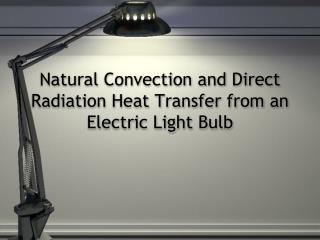DownloadDownload PresentationNatural Convection and Direct Radiation Heat Transfer from an Electric Light Bulb

# Natural Convection and Direct Radiation Heat Transfer from an Electric Light Bulb

Download Presentation## Natural Convection and Direct Radiation Heat Transfer from an Electric Light Bulb

- - - - - - - - - - - - - - - - - - - - - - - - - - - E N D - - - - - - - - - - - - - - - - - - - - - - - - - - -
##### Presentation Transcript

1. Natural Convection and Direct Radiation Heat Transfer from an Electric Light Bulb

2. Aim • Calculate local natural convection heat-transfer coefficients for a sphere • Calculate the local boundary layer • Calculate experimental mean Nusselt number • Compare experimental and theoretical Nusselt numbers

3. Apparatus • Spherical silica glass light bulb with tungsten filament • Eight thermocouples • Voltmeter, • Ammeter • Experimental applied wattages ranged from approximately 5 W to 250W • DO NOT Exceed more than 260 watt

4. Theory • Assumptions made: • True sphere • Voltage delivered to filament is radiated uniformly • Heat flux from wattage absorbed by bulb is of uniform magnitude • Temperature difference between surrounding air and ambient environment approximated by linear function

5. Radiant Heat of the Bulb • A perfect blackbody is a surface that reflects nothing and emits pure thermal radiation. • The Tungsten filament of a light bulb is modeled as a good blackbody radiator. • Because all light from the filament is thermal radiation and almost none of it is reflected from other sources. • The curve for 2,600°C shows that radiation is emitted over the whole range of visible light.

6. The total power emitted as thermal radiation by a blackbody depends on temperature (T) and surface area (A). • The Surface temperature of the filament would be equal to the blackbody temperature if the filament behave as perfect radiator. • However, real surfaces usually emit less than the blackbody power, typically between 10 and 90 percent.

7. The relation between the blackbody temperature and and the surface Temperature could be derived from Stefan-Boltzmann formula Where the Emissivity indicates the deviation of an object from a perfect blackbody radiator It has been determined with an optical pyrometer that when 256 watts is delivered to the tungsten filament that its blackbody temperature TBBr is 3400°R. Since the hemispherical emissivity of tungsten is low (ε = 0.230 Table 2 on labbook) the true temperature of the tungsten coil is

8. You can determine the blackbody temperature at specified power supplied The waves radiated from the filament will either transmit through the glass as light or be absorbed by the glass and lost through radiation and convection. The glass bulb transmits all of the radiation only between 0.35 micron (lower bound) and 2.70 micron (higher bound). By using the Planck’s Law, the fraction of wave transmitted throw the glass could be determined. PlanckRadiation Functions Please see Table 1 Thus the fraction of the watts delivered to the filament, which is transmitted by the glass is: f

9. The remainder fraction of watts which is absorbed by the glass and is lost by convection and radiation from the glass = 1-f Since the glass bulb is modeled as a sphere, so the temperature profile on the surface is symmetric. By knowing the area of the ideal glass bulb (0.315 ft2), the thermal flux leaving the bulb can be determined

10. The flux is transported out both by radiation and natural convection hT = total heat transfer coefficient hT = hc + hR where hc = the convection transfer coefficient and hR = the radiation transfer coefficient T = the localized bulb temperature which is a function of position (in °Rankine ) and T∞ is a the ambient temperature in the laboratory

11. The radiation Heat Transfer Coefficient hR is a function of the difference between the surface temperature of the glass and the ambient temperature and the emissivity of the silica glass. εglass = 0.876 (independent of temperature for all practical purposes) In this experiment, Prandtal Number, Nusselt Number, and Grashofe Number may be calculated. However, to calculate these numbers, 1- It is necessary to determine the mean heat transfer coefficients. This can be done by applying the Simpson’s Rule using the heat transfer coefficient (hC) at several positions on the bulb.

12. 2- Determine the mean air film temperature Tf of the boundary layer Where The mean temperature difference (glass surface temperature-ambient temperature) may be computed by dividing the mean total heat flux by hT obtained from Simpsons

13. Mean Nusselt Number Where r is the radius of the bulb Nusselt number can also be approximated using McAdams equation Gr = Grashof Number = Pr = Prandtl Number =

14. T (T – Tair) Bulb glass Tair Although natural convection is mostly turbulent flow of fluid, But there is always a layer near the surface that is laminar The approximate thickness of the boundary layer may be found by the following equation For local position where k is the thermal conductivity and  is the boundary layer thickness.

15. Increasing temperature yields more radiation (all wavelengths) • Increasing temperature shifts the peak of the energy-flux curve to lower wavelengths/higher frequencies

16. Literature Cited Baum A, Cohen L. 1998. Successful behavioral interventions to prevent cancer: the example of skin cancer. Annual Review of Public Health 19: 319-333. Bird RB, Stewart WE, Lightfoot EN. Transport Phenomena, Wiley, 2002. Chen G. 2003. Nanoscale heat transfer and information technology. Rohsenow Symposium on Future Trends in Heat Transfer at MIT on May 16, 2003. Accessed May 01, 2006 at <http://web.mit.edu/hmtl/www/papers/CHEN.pdf>. Incropera FP, DeWitt DP. Introduction to Heat Transfer, Wiley, 1985. Saddawi, S. 2006. Natural convection and radiation heat transfer from an electric light bulb, Lab Manual, 36-44. Wikipedia. 2005. Black body spectrum as a function of wavelength. Accessed April 30, 2006 at <http://en.wikipedia.org/wiki/Image:Bbs.jpg>.